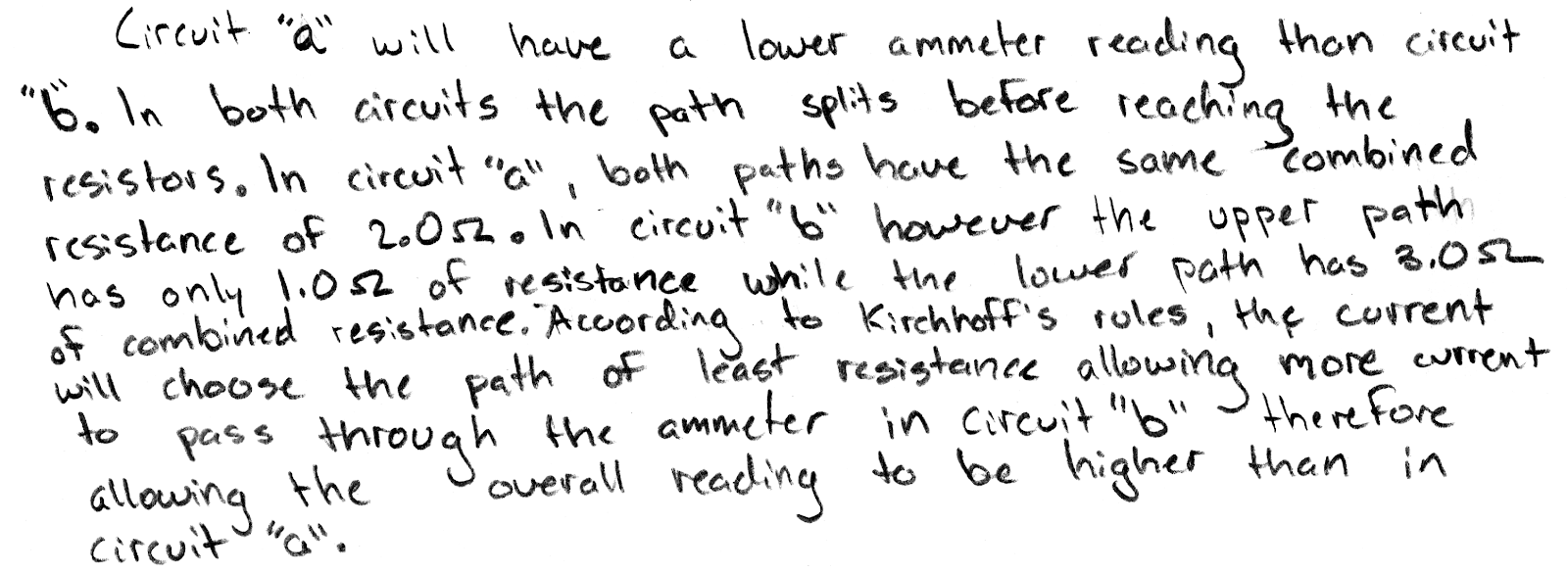## 20180505

### Physics midterm question: comparing ammeter readings

Physics 205B Midterm 2, spring semester 2018
Cuesta College, San Luis Obispo, CATwo separate circuits have an ideal 6.0 emf source connected to three resistors and an ammeter. Discuss which circuit will have a higher ammeter reading (or if there is a tie), and why. Explain your reasoning using Kirchhoff's rules, Ohm's law, and properties of ammeters.

• p:
Correct. Discusses/demonstrates how the ammeter in circuit (a) will have a lower reading than in circuit (b) because:

1. the equivalent resistance of circuit (a) is greater than the equivalent resistance of circuit (b), either by calculating their respective equivalent resistances:

1. Req = [(1/2.0 Ω) + 1/(1.0 Ω + 1.0 Ω)]−1 = 1.0 Ω,

2. Req = [(1/1.0 Ω) + 1/(1.0 Ω + 2.0 Ω)]−1 = 0.75 Ω; and

2. since both circuits have the same emf of 6.0 V, then from Ohm's law, the current in circuit (a) (and its ammeter reading) will be lower than the current in circuit (b):

1. Ⓐ = Icircuit = ΔVeq/Req = (6.0 V)/(1.0 Ω) = 6.0 A,

2. Ⓐ = Icircuit = ΔVeq/Req = (6.0 V)/(0.75 Ω) = 8.0 A.

(May also have recognized that the equivalent resistance of a parallel resistor system must be lower than the lowest single-resistor parallel branch (thus lower than the upper bound of 2.0 Ω for circuit (a), but lower than the upper bound of 1.0 Ω for circuit (b)); and then since the same emf is applied for both circuits (a)-(b), the the circuit with the lower equivalent resistance must have the higher ammeter reading.)

• r:
As (p), but argument indirectly, weakly, or only by definition supports the statement to be proven, or has minor inconsistencies or loopholes.
• t:
Nearly correct, but argument has conceptual errors, or is incomplete.

1. Typically garbles equivalent resistance calculations, for example:

Req = [(1/2.0 Ω) + (1/1.0 Ω) + (1/1.0 Ω)]−1, or

Req = 2.0 Ω + [(1/1.0 Ω) + (1/1.0 Ω)]−1, etc.,

but still recognizes that both circuits have the same emf of 6.0 V, then applies Ohm's law to determine which circuit has more current consistent with their equivalent resistance calculations; or

2. correctly or nearly correctly determines equivalent resistances, but does not clearly carry result into Ohm's law to determine which circuit has more current.

• v:
Limited relevant discussion of supporting evidence of at least some merit, but in an inconsistent or unclear manner. Some attempt at applying properties of Kirchhoff's rules, Ohm's law, and properties of ammeters.
• x:
Implementation/application of ideas, but credit given for effort rather than merit. No clear attempt at systematically applying Kirchhoff's rules, Ohm's law, and properties of ammeters.
• y:
Irrelevant discussion/effectively blank.
• z:
Blank.
Sections 30882, 30883
Exam code: midterm02iFtW
p: 14 students
r: 0 students
t: 16 students
v: 0 students
x: 4 students
y: 0 students
z: 0 students

A sample "p" response (from student 4444):Another sample "p" response (from student 7744):